# 15  Solver

OBJECTIVES

• Application field for Solver.
• Setting up a calculation model.
• Tips using Solver.

Solver is a utility (add-in) within Excel to perform optimization calculations. In such a calculation you want to find an optimal value for a formula in a certain cell, usually a minimum or a maximum and sometimes a very specific value.

objective cell, target cell

This is the cell with a formula for which you want to find the optimal outcome.

variable cells, changing cells, decision variables

These are the cells that determine the outcome of the formula. Changing the values also changes the outcome of the formula.

constraints, restrictions, preconditions

These are restrictions that apply to the variable cells, they often cannot take all possible values and are bound by limits.

Solver has more capabilities than Goal Seek to perform “What-If” analyzes and is much more versatile. Solver adjusts the values of the changing cells within the bounds of the boundary conditions to arrive at the optimal solution of the target cell.

## 15.1 Enabling Solver

The Solver is an add-in and isn’t activated by default through the menu of Excel. If the Solver is not present on the ribbon, then it has to be activated first. This is a one time action.

First, make sure that Solver is available on the ribbon. If so, you can skip this task. Select Data tab, check whether the Analyze group exists and if so whether the Solver is present.

1. Choose File > Options > Add-Ins. A list with Microsoft Office Add-ins is displayed.

2. Select Excel Add-ins in the box Manage.

1. Click on Go…. A list of available Add-ins is displayed.

2. Select the checkbox for Solver Add-in and click on OK.

1. Repeat the check in the first step.

## 15.2 Defining an optimization model

To be able to perform an optimization calculation, you must first set up a suitable calculation model. That is usually much more difficult than using the Solver itself. Making such a model is explained on the basis of the WeatherLeather case.

### 15.2.1 Case description

WeatherLeather, a manufacturer of expensive leather jackets, has two designs created for the new season, a long jacket and a short jacket. The manufacturing of a short jack requires 1 hour on the cutting department and 3 hours on the sewing department. A long jacket requires 2 hours and 4 hours respectively. The availability of labor hours in the cutting department and the sewing department is limited. The available capacity on the cutting department is 32 hours per week and on the sewing department 84 hours a week. The market demand for long leather jackets is limited. No more than 12 long leather jackets can be sold per week. For the short jackets, all jackets made can be sold. There is no production to stock. The profit for a short jacket is $90 and for a long jacket$144.

How many jackets must be made of each type per week to get the highest possible profit?

### 15.2.2 Understanding problem

This step seems so obvious that maybe it seems hardly worth mentioning. It is important to pay attention a little bit more before starting with the formulation of the object cell, variable cells, and restrictions. If you do not fully understand the problem, you risk incorrect formulations of the model. The problem in this example is fairly simple:

How many short and long jackets do you need to produce weekly to get maximum profits, while no more than 32 hours cutting time and 84 hours sewing time is available?

### 15.2.3 Decision variables

Determine the decision variables. These are variables you have determine a value that gives the optimal result. In the Excel model, these are the variable cells. In this example, there are two decision variables which are conveniently referred with a letter:

• $$\mathrm{S}$$ = number of short jackets per week
• $$\mathrm{L}$$ = number of long jackets per week

### 15.2.4 Objective function

You must establish a formula with the optimal value as an outcome. The decision variables are included in this formula. In this example, the objective function is the profit per week and this depends on the number of produced short jackets ($$\mathrm{S}$$) and long jackets ($$\mathrm{L}$$):

$$\mathrm{Profit = 90 \times S + 144 \times L}$$ (This should be maximized.)

In more complex models, it is sometimes difficult to formulate the problem so that one goal function is obtained. It may happen that the decision variables are not directly in the objective function. In such case, the objective function contains variables whose values depend on the decision variables.

### 15.2.5 Constraints

Usually, there are restrictions for the values of the decision variables. These restrictions should be identified and formulated.

In this example, three restrictions that come straight from the case are the limited capabilities for the cutting and sewing department and the market demand for the long jackets.

Besides, there are often general restrictions that have to do with the data type of the decision variables. In this example, the two decision variables, the number of produced jackets, must always have to be positive integers.

This leads to the formulation of the following restrictions:

• Restriction cutting time per week: $$\mathrm{1 \times S + 2 \times L \le 32}$$
• Restriction sewing time per week: $$\mathrm{3 \times S + 4 \times L \le 84}$$
• Restriction market demand long jackets: $$\mathrm{L \le 12}$$
• Restrictions positive values: $$\mathrm{S \ge 0}$$ and $$\mathrm{L \ge 0}$$
• Restrictions integer values: $$\mathrm{S = integer}$$ and $$\mathrm{L = integer}$$

### 15.2.6 Model in Excel

Time to build the model in Excel and solve the problem with the Solver tool. One possible solution you can see in Figure 15.4.

There is not one good way to build this model in Excel. There are just some guidelines. These guidelines are discussed based on Figure 15.4. You can, of course, build the model yourself using the guidelines and the figure, but it is also made available in the file WeatherLeather.xlsx.

### 15.2.7 Guidelines for a Solver model in Excel

1. Organize the data in a logical and orderly manner and put sufficient explanatory text near the cells that contain numbers and formulas. A model is often used in reports later and then it has to be clear what everything is presenting and what it is all about. Highlight the cells with the decision variables (B4 and C4) and the object function (D5) so they are easily recognized. Put the restrictions in a separate part.

2. Put each decision variable in a separate cell and give them a starting value. In the example, the numbers of produced short and long jackets are respectively in cell B4 and cell C4. As starting value 1 is used in both cases. So you can at least verify the correctness of the formulas.

3. Create a formula for the object cell. In D5 the formula is =B5*B4+C5*C4.

4. Create a formula in a separate cell for each restriction. Put in the cell next to it the limit of the restriction.

### 15.2.8 Solver Constraints

The dialog box in Solver with which you add boundary conditions looks like in Figure 15.5.

Every constraint in the Solver consists of three parts: a cell reference, a relationship, and a constraint.

Cell Reference

The cell address or name of the cell range whose values you want to constrain. You can use a single cell or a cell range, but not multiple ranges.

Relationship

Possible operators: <=, =, >=, int, bin, or dif.

• int is displayed as integer later in the model. The values in the cell references must then be integers, within a certain tight tolerance (defined with the Precise of boundary condition in the Solver options).
• bin will be displayed as binary later in the model. The values in the cell references must be either 0 or 1. You can therefore also use this option for “yes/no” decisions.
• dif will be displayed later in the model as AllDifferent. The values in all cell references must be different.
Constraint

A number, a cell reference, a name or a formula that needs to match.

## 15.3 Implementation model

In the case WeatherLeather, the profit must be maximized.

Task 15.2 File: WeatherLeather.xlsx

1. Open the file.

2. Select the object cell D5.

3. Choose tab Data > Solver (group Analyze).

1. Check the following:

• Set Objective: $D$5
• To: Max
2. Click in the box By Changing Variable Cells and select in the worksheet the cell range B4:C4. Excel converts this to $B$4:$C$4.

3. In the restrictions section, click Add. The Add constraint dialog box appears (see Figure 15.5)

4. Add restriction D8 <= E8 and click on OK.

The dialog box Solver Parameters appears again and Excel has the first restriction converted to $D$8 <= $E$8.

2. Add restriction D9 <= E9 and now click on Add.

Now the empty dialog box Add Constraint is displayed. You don’t see now that Excel has added the second restriction.

1. Add restriction D10 <= E10 and click on Add.

2. Add restriction B4:C4 >= 0 and click on Add.

3. Addt restriction B4:C4 int.

4. All restrictions have been added. Click OK.

1. Click on Solve. After a short time, the results pane of the Solver appears.
1. Select Keep Solver Solution (default). Select Answer (section Reports) and then click OK.

The solution found by the Solver is now displayed in the worksheet.

And there has also been created a new worksheet with the name Answer Report ….

• The names that are used in the report are not always accurate or clear. This is because Excel takes these names out of the text cells in the surrounding area. To ensure there are good names in the report, you first have to supply meaningful names to the relevant cells in the worksheet. This is highly recommended.

• At the section Constraints in the Answer Report you can see in the column Status which restrictions are Binding. If so, this indicates that the limit of the constraint is reached and there is no slack. In this example, you can see that all capacity for the cutting time and sewing time is used. For the long jackets, the limit for the market is not reached, there could be 6 more pieces sold.

The problem of WeatherLeather is now solved. A maximum profit of $2664 per week can be achieved by producing 20 short jackets and 6 long jackets. ## 15.4 No solution found. What now? Regularly the Solver reports that no solution could be found: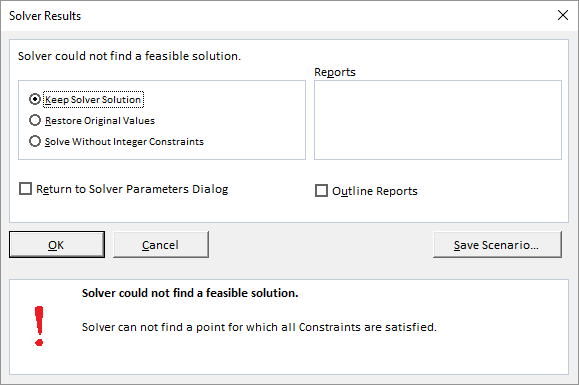Figure 15.11: Message that the solver has not found a viable solution. There are two possibilities now: 1. There is no solution to the formulated problem. 2. There is a solution to the formulated problem, but it is not found by the Solver. In most cases, you don’t know with certainty which situation is present. There are a few guidelines to investigate whether the solution can be found by the Solver. ### 15.4.1 Changing start values variable cells Whether it is possible for the Solver to find a solution or not depends on the starting values of the variable cells, among other things. Experimenting with different starting values can sometimes help to find a solution. ### 15.4.2 Changing Solver options Finding a solution also depends on how the Solver works. The search for a solution follows a so-called iterative process. An iteration is the repeated recalculation of a worksheet until a specific condition is met. The Solver has several settings for this iteration process. Clicking the button Options in the dialog box Solver Parameters displays a window for changing several options of the Solver.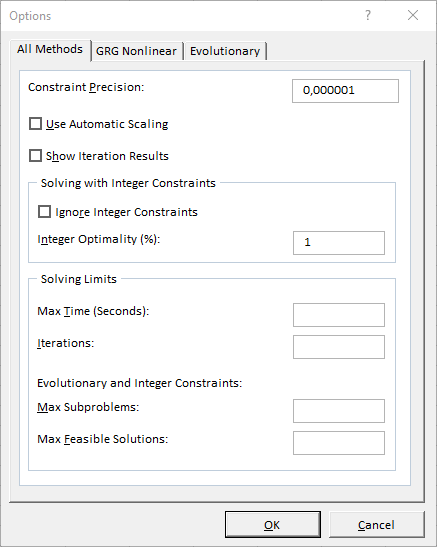Figure 15.12: Options solver. The dialog box offers extensive possibilities for the solution process. Specifications for certain problems can be saved as a model and reloaded. A few of these settings are listed below and discussed shortly. ## 15.5 Exercises Exercise 15.1 Bonbon box (solv001) A well-known bonbon manufacturer wants to offer an assortment box with 2 types of chocolates (cherry bonbons and hazelnut chocolates) and maximum profits. Further is known: • A cherry bonbon takes 16 cm2 space and a hazelnut bonbon 8 cm2. The bonbons are separated by paper. At least 320 cm2 of the box should be covered with bonbons. • A cherry bonbon weighs 2 gram and a hazelnut bonbon weighs 1 gram. Market research has shown that the ideal weight of the contents of the box is between 40 grams and 60 grams. • Also, market research revealed that the box must contain at least 35 bonbons with at least 10 cherry bonbons. • The profit on a cherry bonbon is$0.28 and $0.20 on a hazelnut bonbon. 1. What are the decision variables? 2. What is the objective function? 3. What restrictions are there? 4. Build the model in Excel using the guidelines indicated in this textbook. You see an example in the following figure. Formulas need to be placed in the empty cells. Both decision variables have got a starting value of 10. With these numbers, you can easily check if the formulas are ok and give the correct results.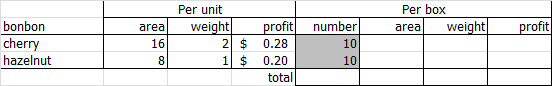1. Enter the model in the Solver and then determine the optimal number of each bonbon type for the box. 1. Two decision variables: number of cherry bonbons per box (= C) and number of hazelnut bonbons per box (= H). 2. Objective function is the maximum profit on a box (= P): P = 0,28*C + 0,20*H 3. Restrictions: • Number of cherry bonbons per box: C >= 10 • Total number of bonbons per box C + H >= 35 • Weight box: 2*C + 1*H >= 40 • Weight box: 2*C + 1*H <= 60 • Area bonbons: 16*C + 8*H >= 320 • Number of bonbons is an integer: C = integer , H = integer • Number of bonbons is a positive number: C >= 0 , H >= 0 Optimal content box: number of cherry chocolates = 10, number of hazelnut chocolates = 40. The profit per box is$10.80 and all constraints are met.

Exercise 15.2 Number of supermarkets (solv002)

A supermarket chain wants to open some new branches for a maximum investment of 14 million dollars. The chain has 4 types of shops: A, B, C, and D. In the following figure, you can see the costs for setting up the four store types and the (expected) sales in the next financial year.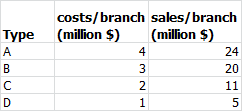All new build branches can be ready for the next financial year. The possible locations are comparable in terms of population. The executive board wants the greatest possible sales of the new branches in the next financial year. How many branches of each type should be built to achieve this?.

1. What are the decision variables?
2. What is the objective function?
3. What restrictions are there?
4. Build the model in Excel using the guidelines indicated in this textbook. You see an example in the following figure. Formulas need to be placed in the empty cells. Both decision variables have got a starting value of 2.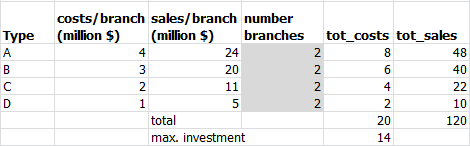1. Enter the model in the Solver and then determine the optimal number of branches of each type and the expected total sales..
1. Four decision variables: Number branches type A (= A), type B (= B), type C (= C) and type D (= D).
2. Objective function is the total sales (= S): S = 24*A + 20*B + 11*C + 5*D
3. Restrictions:
• Maximum investment: 4*A + 3*B + 2*C + 1*D <= 14
• Each number of branches is an integer: A = integer , B = integer , C = integer , D = integer
• Each number of branches is a positive number: A >= 0 , B >= 0 , C >= 0 , D >= 0

Optimal number of branches type A = 0, type B = 4, type C = 1, type D = 0. The total sales is 91 million dollar.

Exercise 15.3 Running shoes (solv003)

A company in Hong Kong manufactures American running shoes. The company produces three models: Runner, Lady T, and Super A. The company wants to make as much profit as possible.

• Manufacturing 1 pair of Lady’s needs 0.31 hour, Runner T needs 0.20 hour and Super A costs 0.25 hour. The maximum number of production hours is 150 hours per week.
• The capital investment per pair for Runner, Lady T, and Super A is respectively $16,$12, and $10. There is$8000 per week available.
• Because of limited machine capacity the production per week is limited to 300 pair Runner, 400 pair Lady T, and 400 pair Super A.
• The profit for Runner is $6 per pair, for Lady T$5 per pair and $4 per pair for Super A. Calculate the optimal production numbers and the maximum profit per week with the Solver. 1. What are the decision variables? 2. What is the objective function? 3. What restrictions are there? 4. Build the model in Excel using the guidelines indicated in this textbook. You see an example in the following figure. Formulas need to be placed in the empty cells. Both decision variables have got a starting value of 100.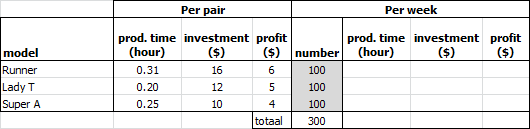1. Enter the model in the Solver and then determine the optimal number of each model and the maximum weekly profit. 1. Three decision variables: Number model Runner per week (= R), number model Lady T per week (= T), and number model Super A per week (= A). 2. Objective function is the maximum profit per week (= P): P = 6*R + 5*T + 4*A 3. Restrictions: • Number model Runner per week: R <= 300 • Number model Lady T per week: T <= 400 • Number model Super A per week: A <= 400 • Maximum production time per week: 0,31*R 0,20*T + 0,25*A <= 150 • Maximum investment per week: 16*R + 12*T +10*A <= 8000 • Number of each model is an integer: R = integer , T = integer , A = integer • Number of each model is a positive number: R >= 0 , T >= 0 en A >= 0 Optimal number Runner = 111, Lady T = 400 and Super A = 142. The profit per week is$3234.

Exercise 15.4 Production of aluminum ladders (solv004)

A manufacturer of aluminum ladders makes three types of ladders. The order in the production is sewing the material first, then assembling and finally the finishing. The production times and profit per ladder are listed in the following figure: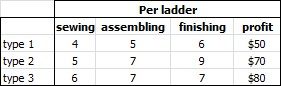The total available production capacity is 80 for sewing, 100 for assembling, and 120 for finishing.

Use the Solver to determine how many ladders of each type should be produced for a maximum profit.

Number type 1 = 0, type 2 = 4 and type 3 = 10. Profit = $1080. Exercise 15.5 Production of dog food (solv005) There are two types of dog food for sale: ordinary and special. A bag of ordinary food contains 3 units minerals, 2 units protein, and 3 units fat. This bag costs$8. A bag special food contains 8 units minerals, 2 units protein, and 1 unit fat. The price of this bag is $14. Over some time a dog should at least consumed 72 units minerals, 36 units protein, and 36 units fat. Determine the quantity of both bags to be bought with minimum costs. Number bags ordinary = 14, special= 4. Costs =$168.

Exercise 15.6 Vitamins for cattle feeding (solv006)

A farmer uses three types of cattle feed, type 1, type 2, and type 3. The nutritional composition and the cost per kg of each type are displayed in the following figure.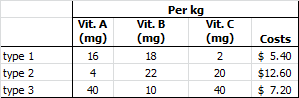A magazine has published the minimum day requirements (MDR) in milligrams per animal for the vitamins A, B, and C. These are 120 mg A, 180 mg B, and 100 mg C. Furthermore, an animal can not eat more than 7½ kg of type 1 and 5 kg of type 2 and 2½ kg of type 3.

How many kilos of each type should the farmer provide every day to get the costs as low as possible and still meet the minimum daily requirements?

Per day: type 1 = 7.500 kg, type 2 = 1.397 kg and type 3 = 1.426 kg. Costs = $68.37. Exercise 15.7 Vitamin pills (solv007) A nutritionist recommends a patient the following minimum day requirements for vitamin B and C: 400 units vitamin B and 800 units vitamin C. The local pharmacy can provide two different vitamin brands: Y and Z. A vitamin pill of brand Y contains 75 units vitamin B and 100 units vitamin C and costs$0.10. A vitamin pill of brand Z contains 50 units vitamin B and 200 units vitamin C and costs $0.08. How much vitamin pills of each branch must be consumed every day at the lowest possible cost? Per day: branch Y = 4 pills and branch Z = 2 pills. Costs =$0.56.

Exercise 15.8 Minimizing transport costs (solv008)

A company has stores in 6 cities (Amersfoort, Eindhoven, Enschede, Lelystad, Tiel, Zwolle). Supplying the stores is done from 3 distribution centers (DC) in Coevorden, Venlo, and Woerden. The stores make every week a sales forecast for the next week and submit the required number of products to the head office. The head office makes a transport planning to the shops based on the available stocks in the DC’s and the required number of products.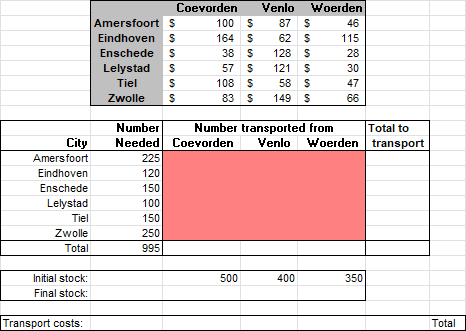Explanation:

• The upper table shows the transport costs per product unit from the DC to the store. So the cost of transport of 1 product from DC Coevorden to the store in Amersfoort is \$100.
• In the column “Number Needed” is the number of products the store needs. So Amersfoort must receive 225 products.
• The area “Number transported from” should display the number of products that will be transported from the DC to the store. The Solver should determine these numbers..
• The row “Initial stock” contains the number of products that are in stock in the DC.

Create the cheapest transport planning using Solver.

File: Solv008.xlsx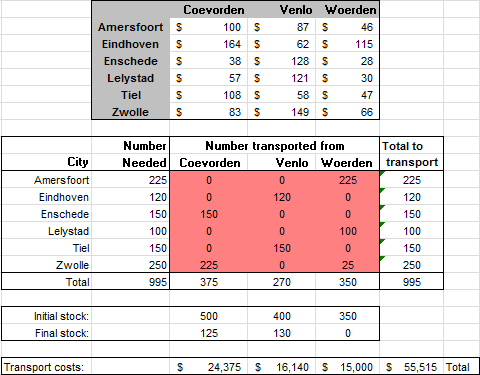Exercise 15.9 Solving system of linear equations (solv009)

Solve the following system of equations using the Solver.

\begin{align*} 6,1x_1 + 4,5x_2 + 5,7x_4 + 7,5x_5 &= 5,7\\ 2,4x_1 + 5,5x_2 - 5,7x_3 + 4,7x_4 + 5,6x_5 &= 5,6\\ 2,4x_1 - 5,0x_2 - 4,6x_3 + 3,6x_4 + 9,7x_5 &= 9,7\\ 4,3x_1 - 5,3x_2 + 3,4x_3 - 8,4x_4 - 5,6x_5 &= -5,6\\ 1,1x_1 - 3,0x_2 + 2,4x_3 - 13x_4 + 3,5x_5 &= 3,5 \end{align*}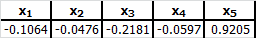Exercise 15.10 Savings bank (solv010)

A savings bank has 3 million euros for investing. The investment portfolios are personal loans, mortgages (first and second mortgages), and car leasing. In the articles of the bank association you can find some conditions regarding the spending of the funds:

• At least 30 percent of the total loaned amount must be invested in mortgages.
• At least 50% of the amount intended for mortgages must be invested in first mortgages.
• Up to 25% of the total loaned amount is allowed to be spent on personal loans and car leasing together.
• Up to 15% of the total loaned amount is allowed to be invested in personal loans.

The annual yield of each type of loan is listed in the following table.

Loan type Yield
Personal loan 18%
First mortgage 12%
Second mortgage 14%
Car leasing 16%

Determine, using the Solver, how much money (in multiples of € 1000,-) in each of the four types of loans should be invested so that the yield is as high as possible.

Loan type Amount
Personal loan 450 K€
First mortgage 1125 K€
Second mortgage 1125 K€
Car leasing 300 K€

Total yield of 422 K€. Rounding on euro cents can cause small differences.